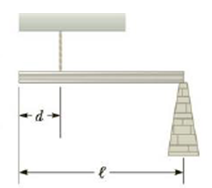Chapter 8, Problem 18P

Chapter
Section
Textbook Problem

A uniform 35.0-kg beam of length ℓ = 5.00 m is supported by a vertical rope located d = 1.20 m from its left end as in Figure P8.18. The right end of the beam is supported by a vertical column. Find (a) the tension in the rope and (b) the force that the column exerts on the right end of the beam.Figure P8.18

(a)

To determine

The tension in the rope.

Explanation

Given info: The mass of the beam is 35.0kg, the length of the beam is 5.00m, the location of the rope from the left end of the beam is 1.20m, and the acceleration due to gravity is 9.80m/s2.

The condition τ=0 about the point O is used for the tension in the rope as follows mg(l/2)T(ld)=0 and this expression is rearranged for T.

The formula for the tension in the rope is,

T=mg(l/2)ld

• m is mass of the beam.
• l is length of the beam.
• d is the location of the rope that attached with the beam from the left end of the beam

(b)

To determine

The force that the column exerts on the right end of the beam.

Still sussing out bartleby?

Check out a sample textbook solution.

See a sample solution

The Solution to Your Study Problems

Bartleby provides explanations to thousands of textbook problems written by our experts, many with advanced degrees!

Get Started

Velocity is a(n) ___ quantity. (2.2)

An Introduction to Physical Science

List three common substances that will sublime.

Chemistry for Today: General, Organic, and Biochemistry

What are genomes?

Human Heredity: Principles and Issues (MindTap Course List)